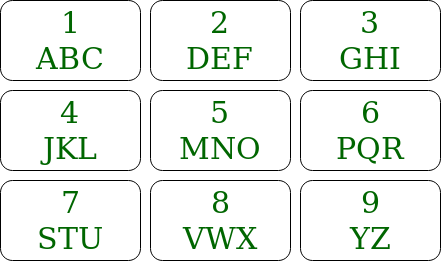# Java ArrayList to print all possible words from phone digits

Given a keypad of a mobile, and keys that need to be pressed, the task is to print all the words which are possible to generate by pressing these numbers.Examples:

```Input: str = "12"
Output: [ad, bd, cd, ae, be, ce, af, bf, cf]
Explanation: The characters that can be formed
by pressing 1 is a, b, c and by pressing 2 characters
d, e, f can be formed.
So all the words will be a combination where first
character belongs to a, b, c and 2nd character belongs
to d, e, f

Input: str = "4"
Output: [j, k, l]
Explanation: The characters that can be formed
by pressing 4 is j, k, l
```

## Recommended: Please try your approach on {IDE} first, before moving on to the solution.

Method 1: Another approach is discussed here Print all possible words from phone digits

Method 2:
Approach: The approach is slightly different from the approach in the other article. Suppose there are n keys which are pressed (a1 a2 a3 ..an). Find all the words that can be formed using (a2 a3 ..an). Suppose 3 characters can be generated by pressing a1 then for every character concatenate the character before all the words and insert them to the list.

For Example:

If the keypress is 12
The characters that can be formed by pressing 1 is a, b, c and by pressing 2 characters d, e, f can be formed.
So all the words that can be formed using 2 are [d, e, f]
So now concatenate ‘a’ with all words returned so, the list is [ad, ae, af] similarly concatenate b and c. So the list becomes [ad, ae, af, bd, be, bf, cd, ce, cf].

Algorithm:

1. Write a recursive function that accepts key press string and returns all the words that can be formed in an Array list.
2. If the length of the given string is 0 then return Arraylist containing empty string.
3. Else recursively call the function with a string except the first character of original string, i.e string containing all the characters from index 1 to n-1. and store the arraylist returned, list and create a new arraylist ans
4. Get the character set of the first character of original string, CSet
5. For every word of the list run a loop through the Cset and concatenate the charcter of Cset infront of the word of list and insert them in the ans arraylist.
6. Return the arraylist, ans.

Implementation:

 `// Java implementation of the approach ` `import` `java.util.ArrayList; ` ` `  `public` `class` `GFG { ` ` `  `    ``// String array to store keypad characters ` `    ``static` `final` `String codes[] ` `        ``= { ``" "``, ``"abc"``, ``"def"``, ` `            ``"ghi"``, ``"jkl"``, ``"mno"``, ` `            ``"pqr"``, ``"stu"``, ``"vwx"``, ` `            ``"yz"` `}; ` ` `  `    ``// Function that returns an Arraylist ` `    ``// which contains all the generated words ` `    ``public` `static` `ArrayList printKeyWords(String str) ` `    ``{ ` ` `  `        ``// If str is empty ` `        ``if` `(str.length() == ``0``) { ` `            ``ArrayList baseRes = ``new` `ArrayList<>(); ` `            ``baseRes.add(``""``); ` ` `  `            ``// Return an Arraylist containing ` `            ``// empty string ` `            ``return` `baseRes; ` `        ``} ` ` `  `        ``// First character of str ` `        ``char` `ch = str.charAt(``0``); ` ` `  `        ``// Rest of the characters of str ` `        ``String restStr = str.substring(``1``); ` ` `  `        ``ArrayList prevRes = printKeyWords(restStr); ` `        ``ArrayList Res = ``new` `ArrayList<>(); ` ` `  `        ``String code = codes[ch - ``'0'``]; ` ` `  `        ``for` `(String val : prevRes) { ` ` `  `            ``for` `(``int` `i = ``0``; i < code.length(); i++) { ` `                ``Res.add(code.charAt(i) + val); ` `            ``} ` `        ``} ` `        ``return` `Res; ` `    ``} ` ` `  `    ``// Driver code ` `    ``public` `static` `void` `main(String[] args) ` `    ``{ ` `        ``String str = ``"23"``; ` ` `  `        ``// Print all the possible words ` `        ``System.out.println(printKeyWords(str)); ` `    ``} ` `} `

Output:

```[dg, eg, fg, dh, eh, fh, di, ei, fi]
```

Complexity Analysis:

• Time Complexity: O(3n).
Though the recursive function runs n times. But the size of the arraylist grows exponentially. So there will be around 3n elements in the arraylist. Therefore, traversing them will take 3n time.
• Space Complexity:O(3n).
Space required to store all words is O(3n). As there will be around 3n words in the output.My Personal Notes arrow_drop_upCheck out this Author's contributed articles.

If you like GeeksforGeeks and would like to contribute, you can also write an article using contribute.geeksforgeeks.org or mail your article to contribute@geeksforgeeks.org. See your article appearing on the GeeksforGeeks main page and help other Geeks.

Please Improve this article if you find anything incorrect by clicking on the "Improve Article" button below.

Improved By : andrew1234